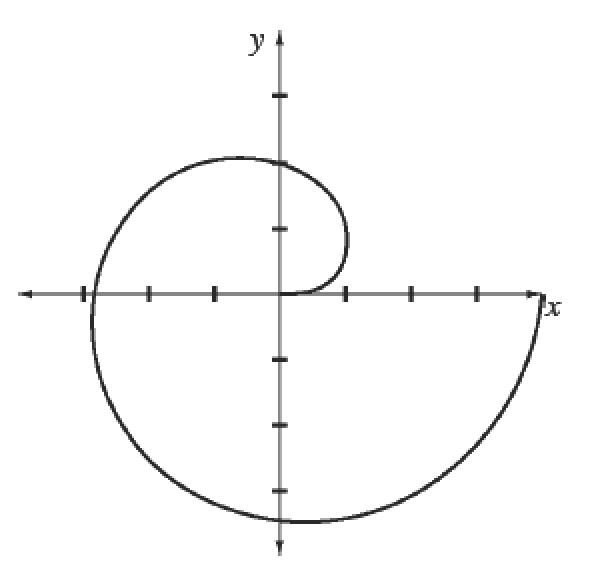### Home > APCALC > Chapter 12 > Lesson 12.2.2 > Problem12-83

12-83.

Multiple Choice: Without a calculator, determine the area of the region in the second and third quadrants bounded by the polar curve $r ( \theta ) = \sqrt { \frac { 8 \theta } { \pi } }$, as shown at right.

1. $4π$

1. $8π$

1. $\frac { 2 \pi } { 3 }(3^{3/2} – 1)$

1. $\frac { 13 \pi ^ { 3 } } { 24 }$

1. $\frac { 18 } { \pi }$$\int_{\pi/2}^{3\pi/2}\frac{1}{2}\cdot\frac{8\theta}{\pi}d\theta$